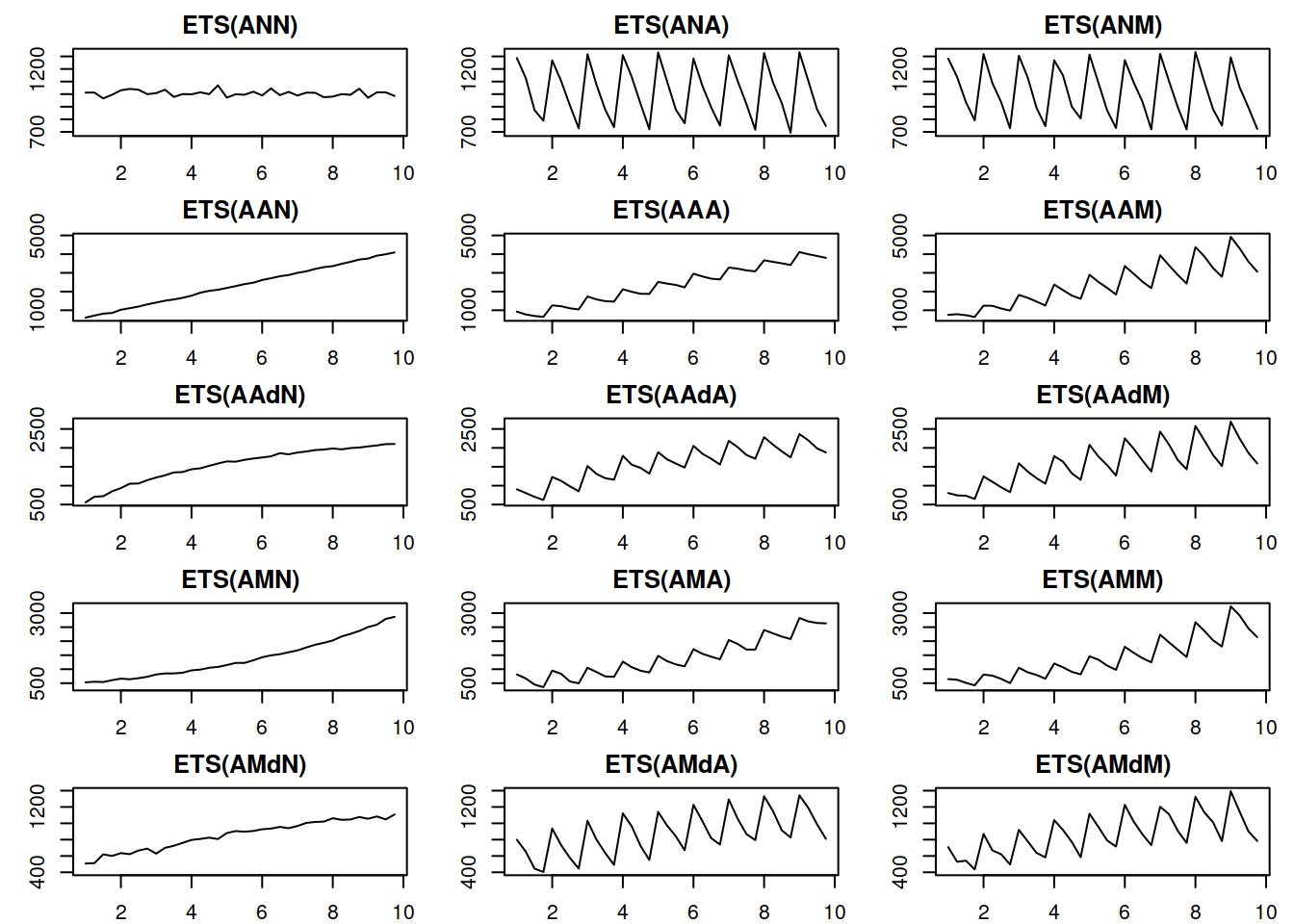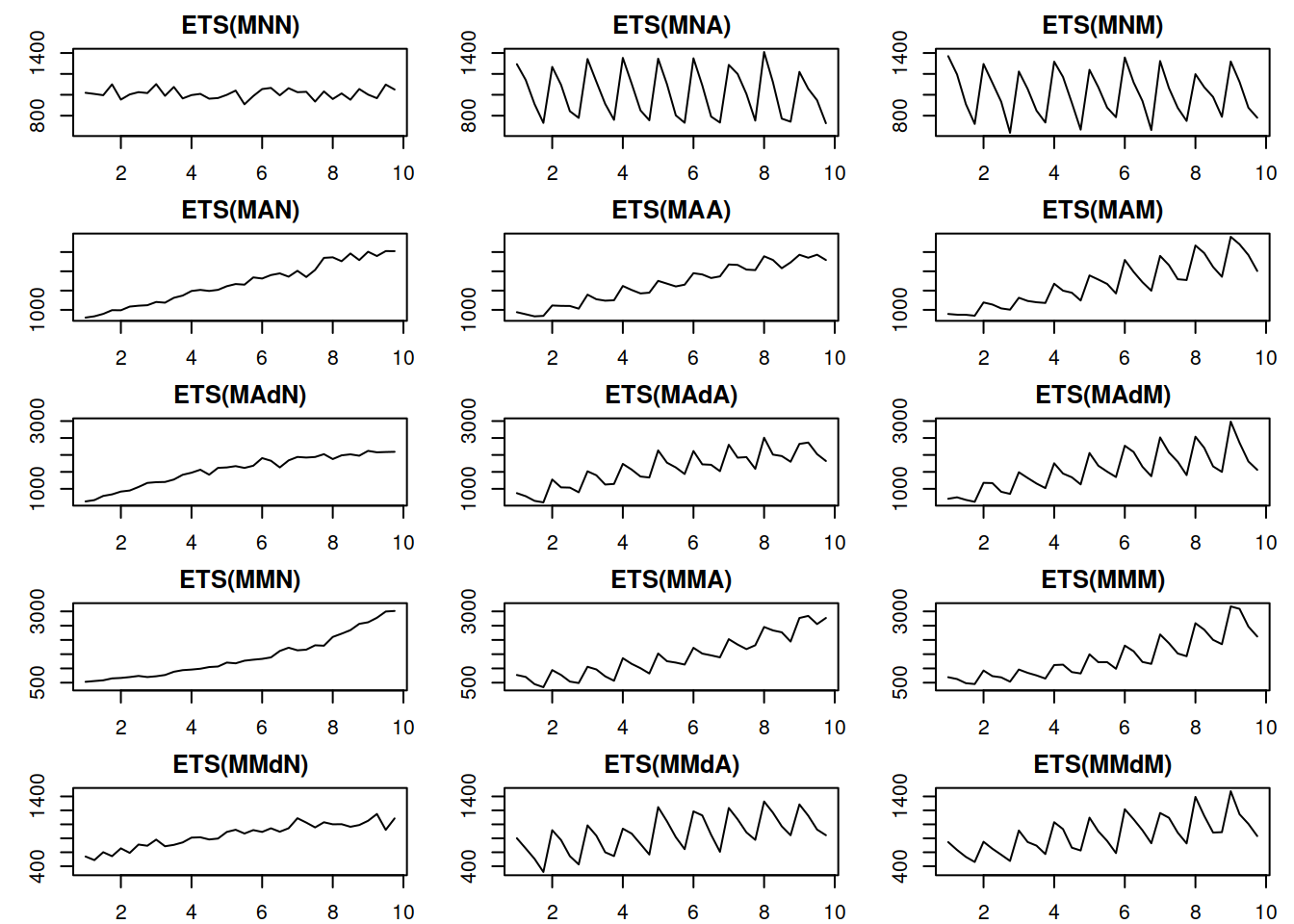This book is in Open Review. I want your feedback to make the book better for you and other readers. To add your annotation, select some text and then click the on the pop-up menu. To see the annotations of others, click the button in the upper right hand corner of the page

## 3.4 ETS taxonomy

Building on the idea of time series components (from Section 3.1) we can move to the ETS taxonomy. ETS stands for “Error-Trend-Seasonality” and defines how specifically the components interact with each other. Based on the type of error, trend and seasonality, proposed a taxonomy, which was then developed further by and refined by . According to this taxonomy, error, trend and seasonality can be:

1. Error: “Additive” (A), or “Multiplicative” (M);
2. Trend: “None” (N), or “Additive” (A), or “Additive damped” (Ad), or “Multiplicative” (M), or “Multiplicative damped” (Md);
3. Seasonality: “None” (N), or “Additive” (A), or “Multiplicative” (M).

According to this taxonomy, the model (3.1) is denoted as ETS(A,A,A) while the model (3.2) is denoted as ETS(M,M,M), and (3.3) is ETS(M,A,M).

The components in ETS taxonomy have clear interpretations. Furthermore, ETS supports 30 models with different types of error, trend and seasonality. Figure 3.10 shows examples of different time series with deterministic (they do not change over time) level, trend, seasonality and with additive error term.Figure 3.10: Time series corresponding to the additive error ETS models

Things to note from the plots in Figure 3.10:

1. When seasonality is multiplicative its amplitude increases with the increase of level of the data while with additive seasonality the amplitude is constant. Compare, for example, ETS(A,A,A) with ETS(A,A,M): the distance between the highest and the lowest points for the former in the first year is roughly the same as in the last year. In the case of ETS(A,A,M) the distance increases with the increase in the level of series.
2. When the trend is multiplicative data exhibits exponential growth / decay result.
3. The damped trend models slow down both additive and multiplicative trends.
4. It is practically impossible to distinguish additive and multiplicative seasonality if the level series does not change because the amplitude of seasonality will be constant in both cases (compare ETS(A,N,A) and ETS(A,N,M)).

Figure 3.11 demonstrates a similar plot for the multiplicative error models.Figure 3.11: Time series corresponding to the multiplicative error ETS models

The plots in Figure 3.11 show roughly the same idea as the additive case, the main difference being that the variance of the error increases with the increase of the level of the data - this becomes clearer on ETS(M,A,N) and ETS(M,M,N) data. This property is called heteroscedasticity in statistics and argue that the main benefit of the multiplicative error models is being able to capture this feature.

In the next chapters we will discuss the most important members of the ETS family. Not all the models in this taxonomy are particularly sensible and some are typically ignored entirely. Although ADAM implements the entire taxonomy we will discuss potential issues and what to expect from them.

### References

• Hyndman, R.J., Koehler, A.B., Ord, J.K., Snyder, R.D., 2008. Forecasting with Exponential Smoothing. Springer Berlin Heidelberg.
• Hyndman, R.J., Koehler, A.B., Snyder, R.D., Grose, S., 2002. A state space framework for automatic forecasting using exponential smoothing methods. International Journal of Forecasting. 18, 439–454. https://doi.org/10.1016/S0169-2070(01)00110-8
• Pegels, C.C., 1969. Exponential Forecasting : Some New Variations. Management Science. 15, 311–315. https://www.jstor.org/stable/2628137